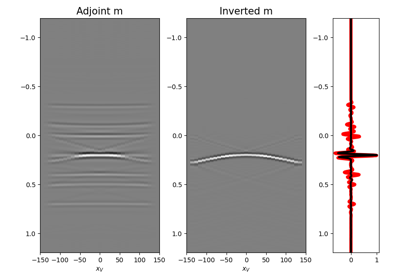# pylops.waveeqprocessing.MDD¶

pylops.waveeqprocessing.MDD(G, d, dt=0.004, dr=1.0, nfmax=None, wav=None, twosided=True, causality_precond=False, adjoint=False, psf=False, dtype='float64', dottest=False, saveGt=True, add_negative=True, smooth_precond=0, fftengine='numpy', **kwargs_solver)[source]

Multi-dimensional deconvolution.

Solve multi-dimensional deconvolution problem using scipy.sparse.linalg.lsqr iterative solver.

Parameters: G : numpy.ndarray Multi-dimensional convolution kernel in time domain of size $$[n_s \times n_r \times n_t]$$ for twosided=False or twosided=True and add_negative=True (with only positive times) or size $$[n_s \times n_r \times 2n_t-1]$$ for twosided=True and add_negative=False (with both positive and negative times) d : numpy.ndarray Data in time domain $$[n_s \,(\times n_{vs}) \times n_t]$$ if twosided=False or twosided=True and add_negative=True (with only positive times) or size $$[n_s \,(\times n_{vs}) \times 2n_t-1]$$ if twosided=True dt : float, optional Sampling of time integration axis dr : float, optional Sampling of receiver integration axis nfmax : int, optional Index of max frequency to include in deconvolution process wav : numpy.ndarray, optional Wavelet to convolve to the inverted model and psf (must be centered around its index in the middle of the array). If None, the outputs of the inversion are returned directly. twosided : bool, optional MDC operator and data both negative and positive time (True) or only positive (False) add_negative : bool, optional Add negative side to MDC operator and data (True) or not (False)- operator and data are already provided with both positive and negative sides. To be used only with twosided=True. causality_precond : bool, optional Apply causality mask (True) or not (False) smooth_precond : int, optional Lenght of smoothing to apply to causality preconditioner adjoint : bool, optional Compute and return adjoint(s) psf : bool, optional Compute and return Point Spread Function (PSF) and its inverse dtype : bool, optional Type of elements in input array. dottest : bool, optional Apply dot-test saveGt : bool, optional Save G and G.H to speed up the computation of adjoint of pylops.signalprocessing.Fredholm1 (True) or create G.H on-the-fly (False) Note that saveGt=True will be faster but double the amount of required memory fftengine : str, optional Engine used for fft computation (numpy, scipy or fftw) **kwargs_solver Arbitrary keyword arguments for chosen solver (scipy.sparse.linalg.cg and pylops.optimization.solver.cg are used as default for numpy and cupy data, respectively) minv : numpy.ndarray Inverted model of size $$[n_r \,(\times n_{vs}) \times n_t]$$ for twosided=False or $$[n_r \,(\times n_vs) \times 2n_t-1]$$ for twosided=True madj : numpy.ndarray Adjoint model of size $$[n_r \,(\times n_{vs}) \times n_t]$$ for twosided=False or $$[n_r \,(\times n_r) \times 2n_t-1]$$ for twosided=True psfinv : numpy.ndarray Inverted psf of size $$[n_r \times n_r \times n_t]$$ for twosided=False or $$[n_r \times n_r \times 2n_t-1]$$ for twosided=True psfadj : numpy.ndarray Adjoint psf of size $$[n_r \times n_r \times n_t]$$ for twosided=False or $$[n_r \times n_r \times 2n_t-1]$$ for twosided=True

MDC
Multi-dimensional convolution

Notes

Multi-dimensional deconvolution (MDD) is a mathematical ill-solved problem, well-known in the image processing and geophysical community .

MDD aims at removing the effects of a Multi-dimensional Convolution (MDC) kernel or the so-called blurring operator or point-spread function (PSF) from a given data. It can be written as

$\mathbf{d}= \mathbf{D} \mathbf{m}$

or, equivalently, by means of its normal equation

$\mathbf{m}= (\mathbf{D}^H\mathbf{D})^{-1} \mathbf{D}^H\mathbf{d}$

where $$\mathbf{D}^H\mathbf{D}$$ is the PSF.

  Wapenaar, K., van der Neut, J., Ruigrok, E., Draganov, D., Hunziker, J., Slob, E., Thorbecke, J., and Snieder, R., “Seismic interferometry by crosscorrelation and by multi-dimensional deconvolution: a systematic comparison”, Geophyscial Journal International, vol. 185, pp. 1335-1364. 2011.

## Examples using pylops.waveeqprocessing.MDD¶09. Multi-Dimensional Deconvolution